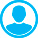APPROXIMATION APPROACH TO THE SOLUTION OF GRAVITY AND MAGNETIC PROBLEMS. 1. THE REGULARIZATION OF THE SYSTEMS OF LINEAR ALGEBRAIC EQUATIONS AS THE MAIN COMPUTATION PROBLEMAbstract (English):
Since no abstract was given for this paper, its subject matter can be derived from the headings given below: Introduction 1.The brief characteristics of the classical theory available for regularizing the systems of linear algebraic equations with approximate data; 2.The method of the regularized expanded systems of second-order linear algebraic equations and some properties of the method of variation regularization; 3.A new theory of regularizing the systems of linear algebraic equations with approximate data; 4.The classification of the main ideas used for constructing and regularizing the systems of linear algebraic equations, developed in terms of this new theory; 5.Conclusion; 6.References.

Keywords:
gravity and magnetic problems, approximation approach, regularization of algebraic equations.